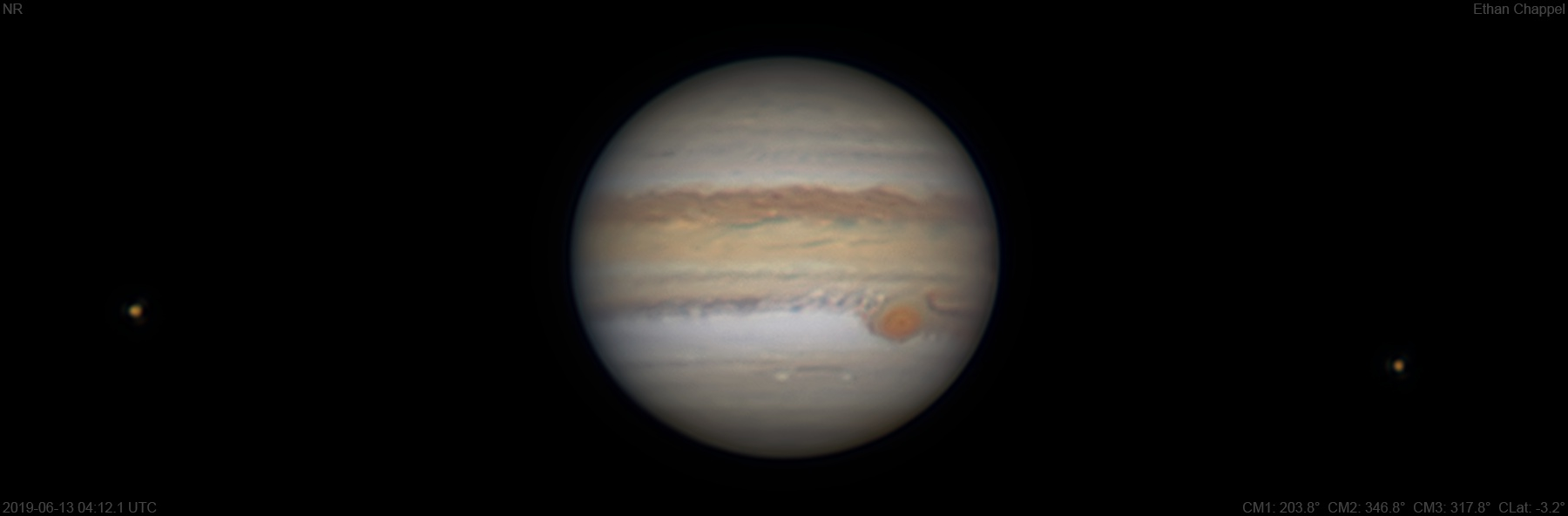# Jupiter 2019-06-13 04:12 UTC

CM1: 203.80°

CM2: 346.80°

CM3: 317.80°

CLat: -3.20°

Description

I decided to setup the C8 even though I wasn't convinced in the moment the sky conditions would be good. The live view was turbulent, but slower than I'm used to seeing. I had both my imaging trains in the same place, so I decided to do a comparison between my ZWO filters for the C8 and my Chroma filters used with the C11 and C14. This image was done through the ZWO filters, while next image will be with the Chroma filters.

Notes:

• White Oval Z is faintly visible on the North Equatorial Belt, north of the Great Red Spot.
• The Equatorial Zone is orange.
• The GRS is off-center in its hollow.
• The GRS hollow is unusually dark.
• South Equatorial Belt clouds are circulating around the GRS and into the South Tropical Zone to the east.
• The SEBs is dark and clumpy.
• Io and Europa appear left and right of the planet respectively.

Equipment

ZWO ASI290MM

Tele Vue 2.5x Powermate

Celestron EdgeHD 8

Logs
```FireCapture v2.6  Settings
------------------------------------
Observer=Ethan Chappel
Camera=ZWO ASI290MM
Filter=G
Profile=Jupiter
Diameter=45.96"
Magnitude=-2.62
CMI=203.7° CMII=346.6° CMIII=317.6°  (during mid of capture)
FocalLength=4100mm (F/14)
Resolution=0.15"
Filename=2019-06-13-0411_9-EC-G-Jup.ser
Date=2019_06_13
Start=04_10_56.750
Mid=04_11_56.757
End=04_12_56.765
Start(UT)=04_10_56.750
Mid(UT)=04_11_56.757
End(UT)=04_12_56.765
Duration=120.015s
Date_format=yyyy_MM_dd
Time_format=HH_mm_ss
LT=UT -6h
Frames captured=6667
File type=SER
Binning=no
Bit depth=8bit
Debayer=no
ROI=1064x406
ROI(Offset)=0x0
FPS (avg.)=55
Shutter=18.00ms
Gain=260 (43%)
FPS=100 (off)
AutoExposure=off
Brightness=1 (off)
HighSpeed=off
AutoHisto=75 (off)
Gamma=50 (off)
USBTraffic=40 (off)
AutoGain=off
SoftwareGain=10 (off)
Histogramm(min)=0
Histogramm(max)=144
Histogramm=56%
Noise(avg.deviation)=0.71
AutoAlign=false
PreFilter=none
Limit=120 Seconds
Sensor temperature=32.7°C
FireCapture v2.6  Settings
------------------------------------
Observer=Ethan Chappel
Camera=ZWO ASI290MM
Filter=R
Profile=Jupiter
Diameter=45.96"
Magnitude=-2.62
CMI=201.4° CMII=344.4° CMIII=315.4°  (during mid of capture)
FocalLength=4050mm (F/14)
Resolution=0.15"
Filename=2019-06-13-0408_2-EC-R-Jup.ser
Date=2019_06_13
Start=04_07_16.809
Mid=04_08_16.810
End=04_09_16.811
Start(UT)=04_07_16.809
Mid(UT)=04_08_16.810
End(UT)=04_09_16.811
Duration=120.002s
Date_format=yyyy_MM_dd
Time_format=HH_mm_ss
LT=UT -6h
Frames captured=5998
File type=SER
Binning=no
Bit depth=8bit
Debayer=no
ROI=1064x406
ROI(Offset)=0x0
FPS (avg.)=49
Shutter=20.00ms
Gain=280 (46%)
FPS=100 (off)
AutoExposure=off
Brightness=1 (off)
HighSpeed=off
AutoHisto=75 (off)
Gamma=50 (off)
USBTraffic=40 (off)
AutoGain=off
SoftwareGain=10 (off)
Histogramm(min)=0
Histogramm(max)=144
Histogramm=56%
Noise(avg.deviation)=0.82
AutoAlign=false
PreFilter=none
Limit=120 Seconds
Sensor temperature=31.0°C
FireCapture v2.6  Settings
------------------------------------
Observer=Ethan Chappel
Camera=ZWO ASI290MM
Filter=B
Profile=Jupiter
Diameter=45.96"
Magnitude=-2.62
CMI=206.4° CMII=349.3° CMIII=320.3°  (during mid of capture)
FocalLength=4100mm (F/14)
Resolution=0.15"
Filename=2019-06-13-0416_3-EC-B-Jup.ser
Date=2019_06_13
Start=04_15_23.243
Mid=04_16_23.244
End=04_17_23.246
Start(UT)=04_15_23.243
Mid(UT)=04_16_23.244
End(UT)=04_17_23.246
Duration=120.003s
Date_format=yyyy_MM_dd
Time_format=HH_mm_ss
LT=UT -6h
Frames captured=5998
File type=SER
Binning=no
Bit depth=8bit
Debayer=no
ROI=1064x406
ROI(Offset)=0x0
FPS (avg.)=49
Shutter=20.00ms
Gain=300 (50%)
FPS=100 (off)
AutoExposure=off
Brightness=1 (off)
HighSpeed=off
AutoHisto=75 (off)
Gamma=50
USBTraffic=40 (off)
AutoGain=off
SoftwareGain=10 (off)
Histogramm(min)=0
Histogramm(max)=128
Histogramm=50%
Noise(avg.deviation)=0.92
AutoAlign=false
PreFilter=none
Limit=120 Seconds
Sensor temperature=33.5°C
```
Measurements
Name Longitude 1 Longitude 2 Longitude 3 Latitude Measurer
GRS F. End 173.9° 316.9° 287.8° -23.6° EGC
GRS P. End 161.1° 304.1° 275.1° -21.9° EGC
GRS N. Edge 168.2° 311.2° 282.2° -17.8° EGC
GRS S. Edge 167.1° 310.1° 281° -27.3° EGC
GRS Center 168.1° 311° 282° -22.8° EGC